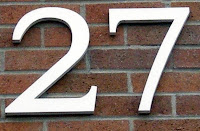## Monday, March 2, 2009

### 27

27 = 33, a perfect cube.

27 is the largest number that is the sum of the digits of its cube: 273 = 19683; 1 + 9 + 6 + 8 + 3 = 27.

27 is the only number that is three times the sum of its digits.

27 is 11011 in base 2 (binary) and 33 in base 8.

27 is the smallest number with two representations as a sum of three squares: 27 = 12 + 12 + 52 = 32 + 32 + 32.

27 is the number of integer partitions of 15 into distinct parts.The chemical element
cobalt has atomic number 27.

Source:
Number Gossip